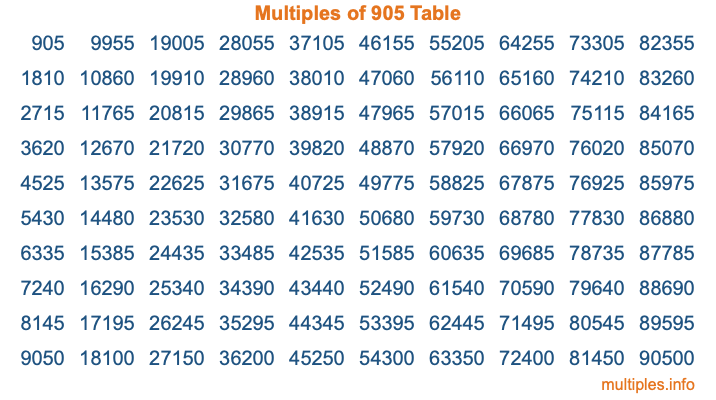Multiples of 905Welcome to the Multiples of 905 page. Here we will first teach you everything you will ever need to know about the multiples of 905, and then give you a study guide summary of everything we taught you to make sure you remember it all. Use this page to look up facts and learn information about the multiples of 905. This page will make you a multiples of nine hundred five expert!

Definition of Multiples of 905
Multiples of 905 are all the numbers that when divided by 905 equal an integer. Each of the multiples of 905 are called a multiple. A multiple of 905 is created by multiplying 905 by an integer.

Therefore, to create a list of multiples of 905, you start with 1 multiplied by 905, then 2 multiplied by 905, then 3 multiplied by 905, and so on for as long as you want. Thus, the list of the first five multiples of 905 is 905, 1810, 2715, 3620, and 4525. To see a larger list of multiples of 905, see the printable image of Multiples of 905 further down on this page. We also have a category where you can choose any nth multiple of 905.

Multiples of 905 Checker
The Multiples of 905 Checker below checks to see if any number of your choice is a multiple of 905. In other words, it checks to see if there is any number (integer) that when multiplied by 905 will equal your number. To do that, we divide your number by 905. If the the quotient is an integer, then your number is a multiple of 905.

Is  a multiple of 905?

Least Common Multiple of 905 and ...
A Least Common Multiple (LCM) is the lowest multiple that two or more numbers have in common. This is also called the smallest common multiple or lowest common multiple and is useful to know when you are adding our subtracting fractions. Enter one or more numbers below (905 is already entered) to find the LCM.

Check out our LCM Calculator if you need more details about the Least Common Multiple or if you need the LCM for different numbers for adding and subtraction fractions.

nth Multiple of 905
As we stated above, 905 is the first multiple of 905, 1810 is the second multiple of 905, 2715 is the third multiple of 905, and so on. Enter a number below to find the nth multiple of 905.

th multiple of 905

Multiples of 905 vs Factors of 905
905 is a multiple of 905 and a factor of 905, but that is where the similarities end. All postive multiples of 905 are 905 or greater than 905. All positive factors of 905 are 905 or less than 905.

Below is the beginning list of multiples of 905 and the factors of 905 so you can compare:

Multiples of 905: 905, 1810, 2715, 3620, 4525, etc.

Factors of 905: 1, 5, 181, 905

As you can see, the multiples of 905 are all the numbers that you can divide by 905 to get a whole number. The factors of 905, on the other hand, are all the whole numbers that you can multiply by another whole number to get 905.

It's also interesting to note that if a number (x) is a factor of 905, then 905 will also be a multiple of that number (x).

Multiples of 905 vs Divisors of 905
The divisors of 905 are all the integers that 905 can be divided by evenly. Below is a list of the divisors of 905.

Divisors of 905: 1, 5, 181, 905

The interesting thing to note here is that if you take any multiple of 905 and divide it by a divisor of 905, you will see that the quotient is an integer.

Multiples of 905 Table
Below is an image of the first 100 multiples of 905 in a table. The table is in chronological order, column by column. The first column has the first ten multiples of 905, the second column has the next ten multiples of 905, and so on.The Multiples of 905 Table is also referred to as the 905 Times Table or Times Table of 905. You are welcome to print out our table for your studies.

Negative Multiples of 905
Although not often discussed or needed in math, it is worth mentioning that you can make a list of negative multiples of 905 by multiplying 905 by -1, then by -2, then by -3, and so on, to get the following list of negative multiples of 905:

-905, -1810, -2715, -3620, -4525, etc.

Multiples of 905 Summary
Below is a summary of important Multiples of 905 facts that we have discussed on this page. To retain the knowledge on this page, we recommend that you read through the summary and explain to yourself or a study partner why they hold true.

There are an infinite number of multiples of 905.

A multiple of 905 divided by 905 will equal a whole number.

905 divided by a factor of 905 equals a divisor of 905.

The nth multiple of 905 is n times 905.

The largest factor of 905 is equal to the first positive multiple of 905.

905 is a multiple of every factor of 905.

905 is a multiple of 905.

A multiple of 905 divided by a divisor of 905 equals an integer.

905 divided by a divisor of 905 equals a factor of 905.

Any integer times 905 will equal a multiple of 905.

Multiples of a Number
Here you can get the multiples of another number, all with the same attention to detail as we did for multiples of 905 on this page.

Multiples of
Multiples of 906
Did you find our page about multiples of nine hundred five educational? Do you want more knowledge? Check out the multiples of the next number on our list!